{{announcement.body}}
{{announcement.title}}

# Drowsy Detection Using Facial Landmarks Extraction and Deep Neural Networks

DZone 's Guide to

# Drowsy Detection Using Facial Landmarks Extraction and Deep Neural Networks

### How can you detect a drowsy person using facial landmarks as an input of a neural network?

· AI Zone ·
Free Resource

Comment (1)

Save
{{ articles.views | formatCount}} Views

## Introduction

The goal of this article is to explain how you can detect a drowsy person using facial landmarks as an input of a neural network, a 3D convolutional neural network, in this case, to sound an alarm to awake the user and to prevent some kind of accident.

The idea is to extract a group of frames from a webcam and then extract from them the facial landmarks, specifically the position of both eyes, then pass these coordinates to the neural model to get a final classification which will tell us if the user is awake, or falling sleep.

## Methodology

Recent works have shown that activity recognition can be achieved with 3D convolutional neural networks or Conv3D because of the capacity of analyzing not a single frame but a group of them, this group of frames are a short video where the activity is contained.

Having said that and considering drowsy as an activity that can be contained in a video, it makes sense to use Conv3D to try to predict drowsiness.

The first step is to extract a frame from a camera, in our case a webcam. Once we have the frame, we use a python library called dlib where a facial landmark detector is included; the result is a collection of x, y coordinates which indicate where the facial landmarks are.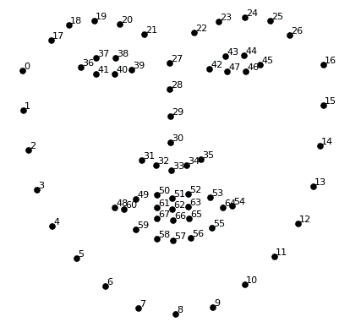Figure 1: Facial Landmarks

Even when we get a collection of points, we are only interested in the position of the eyes, so we are going to keep only the twelve points that belong to the eyes.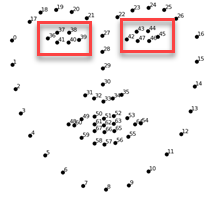Figure 2: Region of interest of the facial landmarks

Until now, we have the facial landmarks of a single frame. Nevertheless, we want to give our system the sense of the sequence, and to do so, we are not considering single frames to make our final prediction, we better take a group of them.

We consider that analyzing one second of video at a time is enough to make good drowsiness predictions. Hence, we keep ten facial landmarks detection, which is equivalent of one second of video; then, we concatenate them in one single pattern that is an array with the shape (10, 12, 12); 10 frames with 12 points in x-coordinates and 12 points in y-coordinates. This array is the input of our Conv3D model to get the final classification.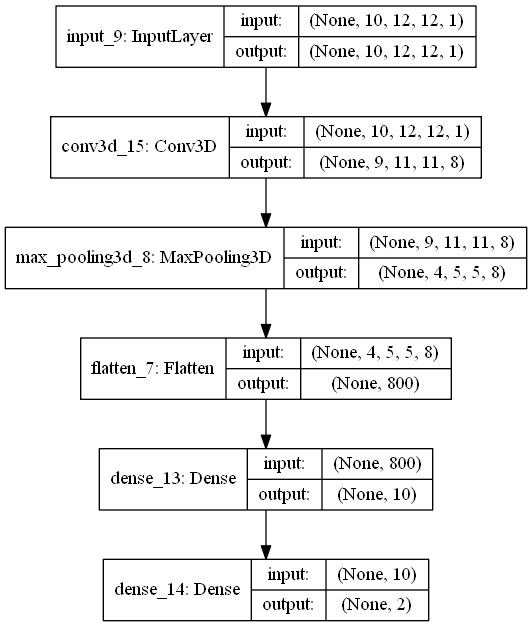Figure 3: Neural Model

The first hidden layer of our model is a 3D convolutional layer, followed by a max pooling layer and a flatten layer, which results in a vector of eight hundred neurons. The next layer is a dense layer of teen units, with relu activation function.  The last layer of the model y is composed of two neurons where the activation function is a softmax function, composed of two neurons, one per class.

## Architecture

The webcam is always streaming video, but we analyze a single frame every 0.1 seconds until we reach 10 samples, the equivalent of 1 second, to extract the facial landmarks and keep only the points corresponding to both eyes. We group the points with an overlapping of 7 units, which means that we group the points from frame one to ten, the next group is formed from the point of the frame four to the frame thirteen.

Once we have a group of eyes’ points (x, y coordinates) we pass them to our neural model to get a classification, whose result can be [1, 0] which represents “awake”, or [0, 1] that represents “drowsy”. In other words, we are analyzing the streaming of the webcam in small chunks to get a prediction of drowsiness each second.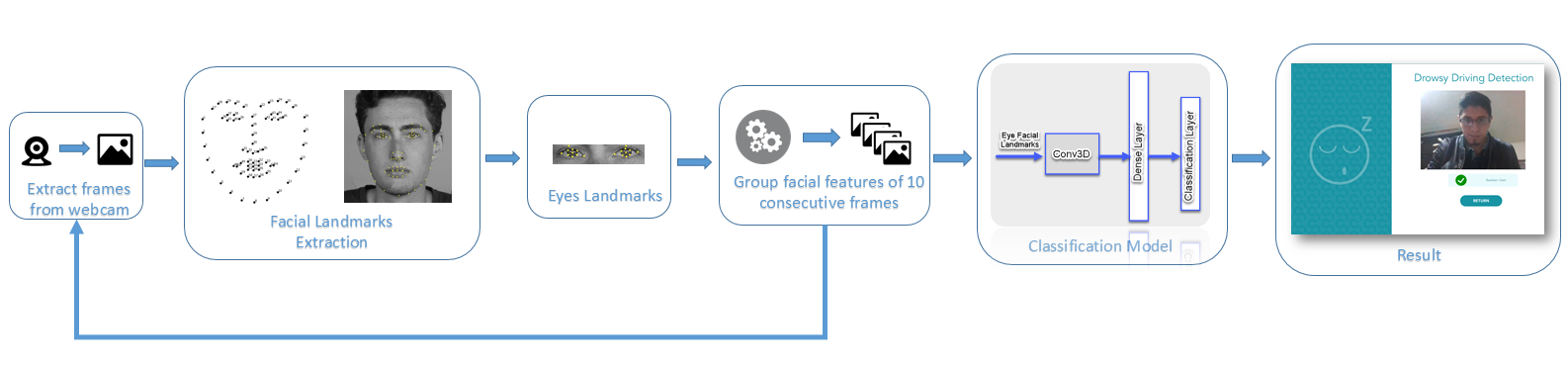Figure 4: Solution Architecture

## Implementation

Here, you can find how each of the elements used for this article was obtained.

• The system was implemented on python 3.5
• The extraction of frames from the webcam was achieved using OpenCV for python
• The facial landmarks were extracted with the library dlib
• The model was constructed using keras
• The front-end was deployed with the help of flask.
``````from keras.utils import plot_model
from keras.models import Model
from keras.layers import Input
from keras.layers import Dense
from keras.layers import Flatten
from keras.layers.convolutional import Conv3D
from keras.layers.pooling import MaxPooling3D
from keras.models import Sequential

visible = Input(shape=(10,12,12,1))
conv1 = Conv3D(8, kernel_size=2, activation='relu')(visible)
pool1 = MaxPooling3D(pool_size=(2, 2, 2))(conv1)
flat1 = Flatten()(pool1)
hidden1 = Dense(10, activation='relu')(flat1)
output = Dense(2, activation='softmax')(hidden1)
model = Model(inputs=visible, outputs=output)``````

## Results

We trained our final model only for two hundred epochs, the optimizer used for the training of this model was ADAM. Of course, we tried with many other models, but the best result is the one shown here.

The final result of this work is a front-end where the user’s webcam is shown. The streaming is analyzed every one second, and the prediction “drowsy” or “awake” is shown under the video.  As an additional feature in our final example, if the user is detected as “drowsy,” the system fires a sound alarm.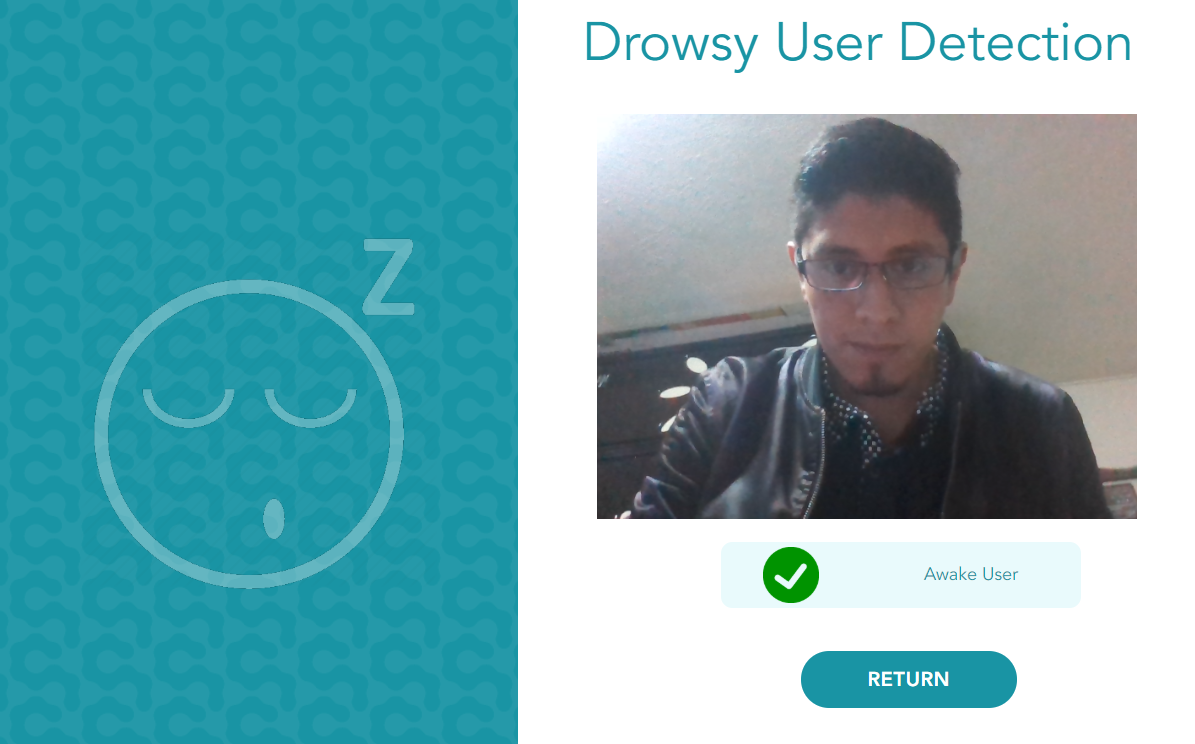Figure 5: Front-End Result

For further experiments, the solution proposed here can easily be extended to work on a smartphone or even in an embedded system running an Ubuntu distribution such as the well-known raspberry pi.

Topics:
ai ,ai tutorial ,computer vision ,deep learning ,deep neural networks

Comment (1)

Save
{{ articles.views | formatCount}} Views

Opinions expressed by DZone contributors are their own.